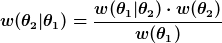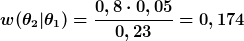# Why is Bayes' theorem important

## Bayes' theorem

1. term: Theorem from the calculus of probabilities for calculating conditional probabilities. Denote w (θ1) and w (θ2) the unconditional probabilities for the occurrence of the stochastically dependent events θ1 and θ2, and further denotes w (θ2│θ1) the (conditional) probability for the event θ2 on condition that θ1 has occurred, the inverse conditional probability w (θ1│θ2) according to Bayes' theorem:2. application: The Bayes' theorem is especially used in information processing (see also information value): Denotes θ2 a certain event (e.g. future bankruptcy of a borrower) and θ1 information about the event (e.g. credit check by the lender), then the adjustment of the probability judgment about θ follows2 (in the example: adjustment of the probability judgment about the bankruptcy of the borrower) rationally according to Bayes' theorem. The a priori judgment w (θ2) into the a posteriori judgment w (θ1) converted according to the formula given.

3. Calculation example: A lender estimates the likelihood that a borrower will become insolvent (event θ2), to 5 percent. He also assumes that the credit check routinely carried out in-house provides a correct assessment with an 80 percent probability. The test turns out negative (event θ1). The probability for this negative test result is w (θ1) = 0.8 x 0.05 + 0.2 x 0.95 = 0.23. It is made up of two individual probabilities: the probability of a correct insolvency forecast (0.8 · 0.05) plus the probability of an incorrect insolvency forecast (0.2 · 0.95). According to Bayes' theorem, the lender adjusts its probability judgment as follows:The probability of insolvency increases from an initial 5 percent (a priori judgment) to 17.4 percent (a posteriori judgment).